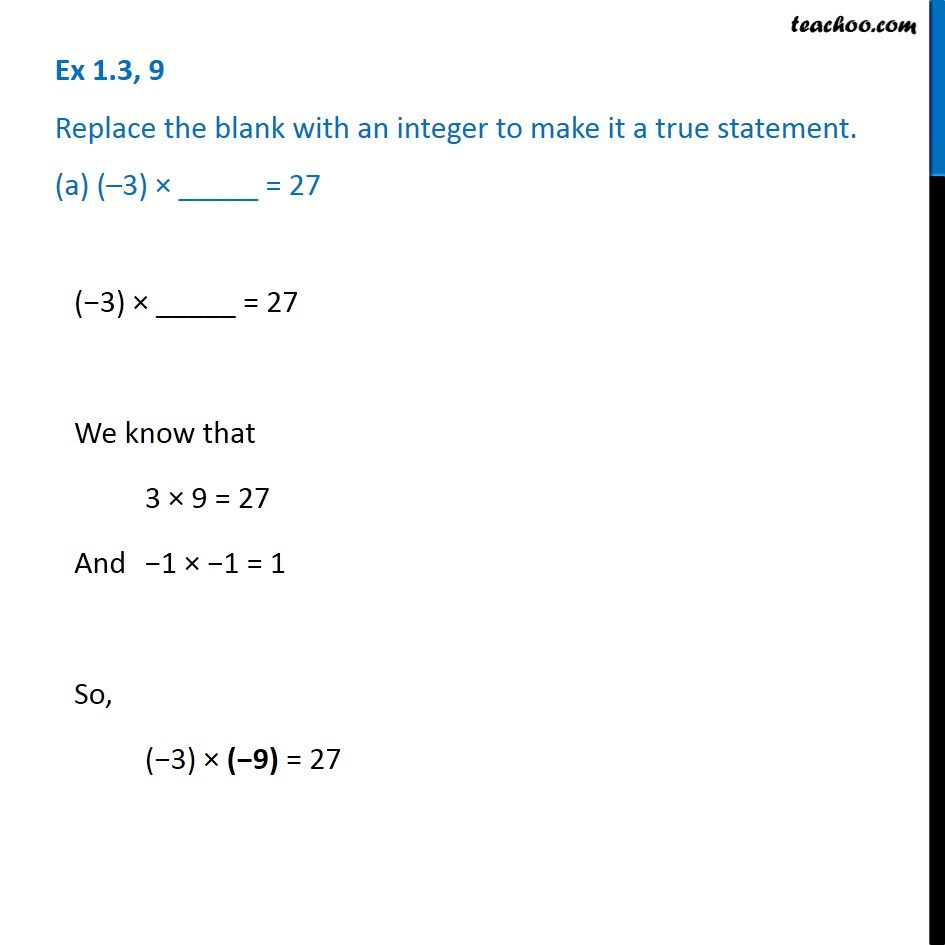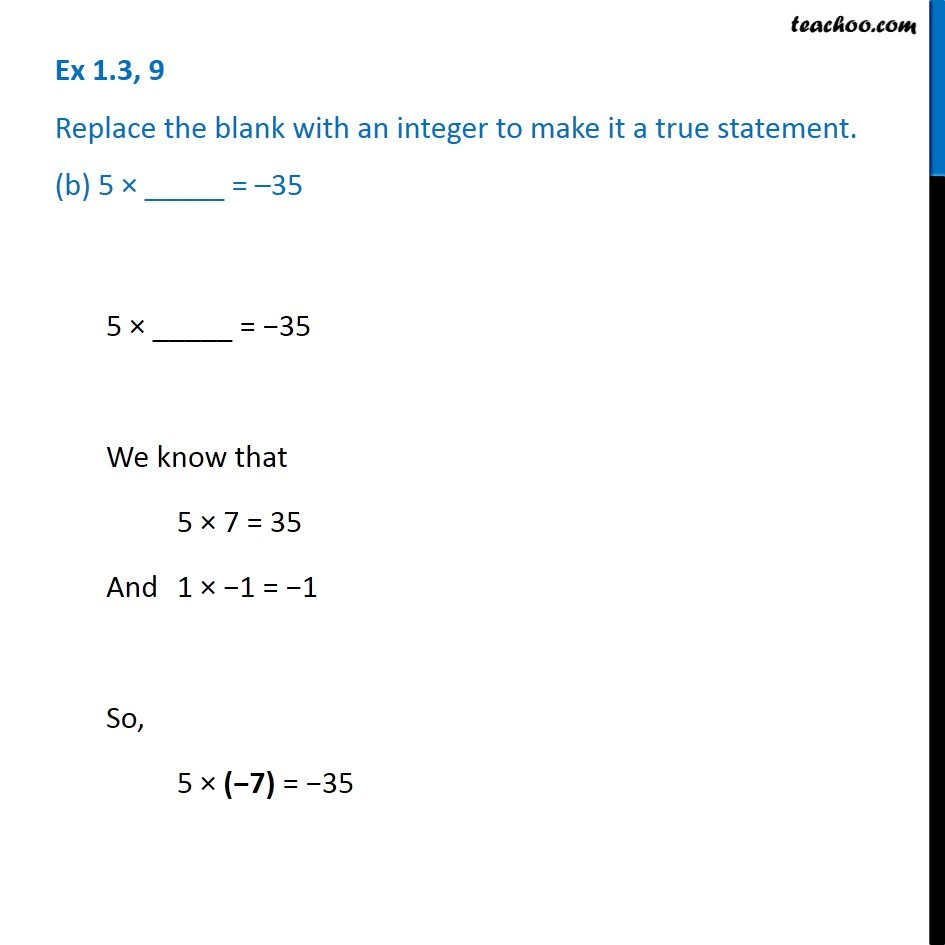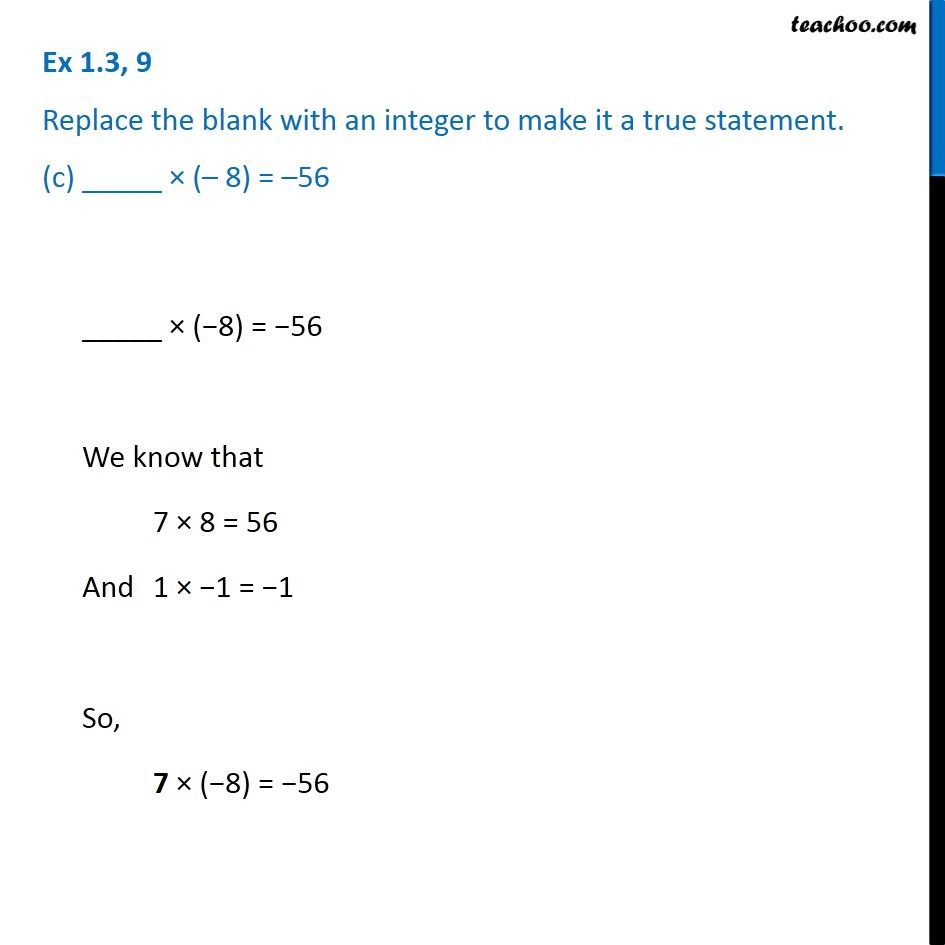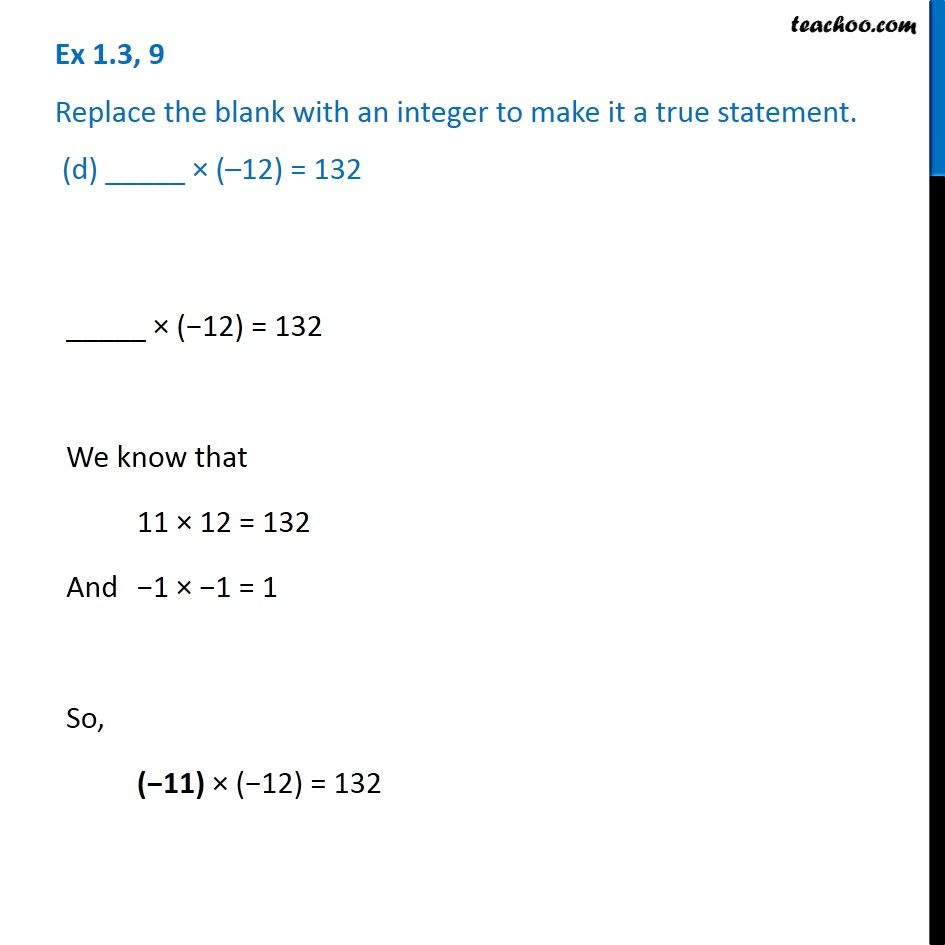1. Chapter 1 Class 7 Integers
2. Concept wise
3. Multiplication of Integers

Transcript

Ex 1.3, 9 Replace the blank with an integer to make it a true statement. (a) (–3) × _____ = 27 (−3) × _____ = 27 We know that 3 × 9 = 27 And −1 × −1 = 1 So, (−3) × (−9) = 27 Ex 1.3, 9 Replace the blank with an integer to make it a true statement. (b) 5 × _____ = –35 5 × _____ = −35 We know that 5 × 7 = 35 And 1 × −1 = −1 So, 5 × (−7) = −35 Ex 1.3, 9 Replace the blank with an integer to make it a true statement. (c) _____ × (– 8) = –56 _____ × (−8) = −56 We know that 7 × 8 = 56 And 1 × −1 = −1 So, 7 × (−8) = −56 Ex 1.3, 9 Replace the blank with an integer to make it a true statement. (d) _____ × (–12) = 132 _____ × (−12) = 132 We know that 11 × 12 = 132 And −1 × −1 = 1 So, (−11) × (−12) = 132